### I just announced the new Learn Spring course, focused on the fundamentals of Spring 5 and Spring Boot 2:

>> CHECK OUT THE COURSE

## 1. Overview

Most of the time, when we're implementing graph-based algorithms, we also need to implement some utility functions.

JGraphT is an open-source Java class library which not only provides us with various types of graphs but also many useful algorithms for solving most frequently encountered graph problems.

In this article, we’ll see how to create different types of graphs and how convenient it is to use the provided utilities.

## 2. Maven Dependency

Let's start by adding the dependency to our Maven project:

``````<dependency>
<groupId>org.jgrapht</groupId>
<artifactId>jgrapht-core</artifactId>
<version>1.0.1</version>
</dependency>``````

## 3. Creating Graphs

JGraphT supports various types of graphs.

### 3.1. Simple Graphs

For starters, let’s create a simple graph with a vertex of type String:

``````Graph<String, DefaultEdge> g
= new SimpleGraph<>(DefaultEdge.class);

### 3.2. Directed/Undirected Graphs

It also allows us to create directed/undirected graphs.

In our example, we’ll create a directed graph and use it to demonstrate other utility functions and algorithms: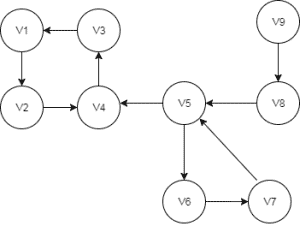``````DirectedGraph<String, DefaultEdge> directedGraph
= new DefaultDirectedGraph<>(DefaultEdge.class);
// Add remaining vertices and edges``````

### 3.3. Complete Graphs

Similarly, we can also generate a complete graph: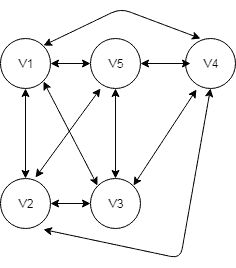``````public void createCompleteGraph() {
completeGraph = new SimpleWeightedGraph<>(DefaultEdge.class);
CompleteGraphGenerator<String, DefaultEdge> completeGenerator
= new CompleteGraphGenerator<>(size);
VertexFactory<String> vFactory = new VertexFactory<String>() {
private int id = 0;
public String createVertex() {
return "v" + id++;
}
};
completeGenerator.generateGraph(completeGraph, vFactory, null);
}``````

### 3.4. Multi-Graphs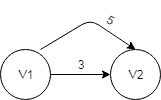Other than simple-graphs, API also provides us with multigraphs (graphs with multiple paths between two vertices).

Besides, we can have weighted/unweighted or user-defined edges in any graph.

Let's create a multigraph with weighted edges:

``````public void createMultiGraphWithWeightedEdges() {
multiGraph = new Multigraph<>(DefaultWeightedEdge.class);
multiGraph.setEdgeWeight(edge1, 5);

multiGraph.setEdgeWeight(edge2, 3);
}``````

In addition to this, we can have unmodifiable (read-only) and listenable (allows external listeners to track modifications) graphs as well as subgraphs. Also, we can always create all compositions of these graphs.

Further API details can be found here.

## 4. API Algorithms

Now, that we've got full fledge graph objects, let’s look at some common problems and their solutions.

### 4.1. Graph Iteration

We can traverse the graph using various iterators such as BreadthFirstIterator, DepthFirstIterator, ClosestFirstIterator, RandomWalkIterator as per the requirement.
We simply need to create an instance of respective iterators by passing graph objects:

``````DepthFirstIterator depthFirstIterator
= new DepthFirstIterator<>(directedGraph);

Once we get the iterator objects, we can perform the iteration using hasNext() and next() methods.

### 4.2. Finding the Shortest Path

It provides implementations of various algorithms such as Dijkstra, Bellman-Ford, Astar, and FloydWarshall in the org.jgrapht.alg.shortestpath package.

Let's find the shortest path using Dijkstra’s algorithm:

``````@Test
public void whenGetDijkstraShortestPath_thenGetNotNullPath() {
DijkstraShortestPath dijkstraShortestPath
= new DijkstraShortestPath(directedGraph);
List<String> shortestPath = dijkstraShortestPath
.getPath("v1","v4").getVertexList();

assertNotNull(shortestPath);
}``````

Similarly, to get the shortest path using the Bellman-Ford algorithm:

``````@Test
public void
whenGetBellmanFordShortestPath_thenGetNotNullPath() {
BellmanFordShortestPath bellmanFordShortestPath
= new BellmanFordShortestPath(directedGraph);
List<String> shortestPath = bellmanFordShortestPath
.getPath("v1", "v4")
.getVertexList();

assertNotNull(shortestPath);
}``````

### 4.3. Finding Strongly Connected Subgraphs

Before we get into the implementation, let’s briefly look at what strongly connected subgraphs mean. A subgraph is said to be strongly connected only if there is a path between each pair of its vertices.

In our example, {v1,v2,v3,v4} can be considered a strongly connected subgraph if we can traverse to any vertex irrespective of what the current vertex is.

We can list four such subgraphs for the directed graph shown in the above image:
{v9},{v8},{v5,v6,v7},{v1,v2,v3,v4}

Implementation to list out all strongly connected subgraphs:

``````@Test
public void
whenGetStronglyConnectedSubgraphs_thenPathExists() {

StrongConnectivityAlgorithm<String, DefaultEdge> scAlg
= new KosarajuStrongConnectivityInspector<>(directedGraph);
List<DirectedSubgraph<String, DefaultEdge>> stronglyConnectedSubgraphs
= scAlg.stronglyConnectedSubgraphs();
List<String> stronglyConnectedVertices
= new ArrayList<>(stronglyConnectedSubgraphs.get(3)
.vertexSet());

String randomVertex1 = stronglyConnectedVertices.get(0);
String randomVertex2 = stronglyConnectedVertices.get(3);
AllDirectedPaths<String, DefaultEdge> allDirectedPaths
= new AllDirectedPaths<>(directedGraph);

List<GraphPath<String, DefaultEdge>> possiblePathList
= allDirectedPaths.getAllPaths(
randomVertex1, randomVertex2, false,
stronglyConnectedVertices.size());

assertTrue(possiblePathList.size() > 0);
}``````

### 4.4. Eulerian Circuit

A Eulerian Circuit in a graph G is a circuit that includes all vertices and edges of G. A graph which has it is a Eulerian Graph.

Let's have a look at the graph: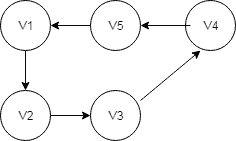``````public void createGraphWithEulerianCircuit() {
SimpleWeightedGraph<String, DefaultEdge> simpleGraph
= new SimpleWeightedGraph<>(DefaultEdge.class);
IntStream.range(1,5)
IntStream.range(1,5)
.forEach(i-> {
int endVertexNo = (i + 1) > 5 ? 1 : i + 1;
});
}``````

Now, we can test whether a graph contains Eulerian Circuit using the API:

``````@Test
public void givenGraph_whenCheckEluerianCycle_thenGetResult() {
HierholzerEulerianCycle eulerianCycle
= new HierholzerEulerianCycle<>();

assertTrue(eulerianCycle.isEulerian(simpleGraph));
}
@Test
public void whenGetEulerianCycle_thenGetGraphPath() {
HierholzerEulerianCycle eulerianCycle
= new HierholzerEulerianCycle<>();
GraphPath path = eulerianCycle.getEulerianCycle(simpleGraph);

assertTrue(path.getEdgeList()
.containsAll(simpleGraph.edgeSet()));
}``````

### 4.5. Hamiltonian Circuit

A GraphPath that visits each vertex exactly once is known as Hamiltonian Path.

A Hamiltonian cycle (or Hamiltonian circuit) is a Hamiltonian Path such that there is an edge (in the graph) from the last vertex to the first vertex of the path.

We can find optimal Hamiltonian Cycle for complete graph with HamiltonianCycle.getApproximateOptimalForCompleteGraph() method.

This method will return an approximate minimal traveling salesman tour (Hamiltonian cycle). The optimal solution is NP-complete, so this is a decent approximation that runs in polynomial time:

``````public void
whenGetHamiltonianCyclePath_thenGetVerticeSequence() {
List<String> verticeList = HamiltonianCycle
.getApproximateOptimalForCompleteGraph(completeGraph);

assertEquals(verticeList.size(), completeGraph.vertexSet().size());
}``````

### 4.6. Cycle Detector

We can also check if there are any cycles in the graph. Currently, CycleDetector only supports directed graphs:

``````@Test
public void whenCheckCycles_thenDetectCycles() {
CycleDetector<String, DefaultEdge> cycleDetector
= new CycleDetector<String, DefaultEdge>(directedGraph);

assertTrue(cycleDetector.detectCycles());
Set<String> cycleVertices = cycleDetector.findCycles();

assertTrue(cycleVertices.size() > 0);
}``````

## 5. Graph Visualization

JGraphT allows us to generate visualizations of graphs and save them as images, first let's add the jgrapht-ext extension dependency from Maven Central:

``````<dependency>
<groupId>org.jgrapht</groupId>
<artifactId>jgrapht-ext</artifactId>
<version>1.0.1</version>
</dependency>``````

Next, let's create a simple directed graph with 3 vertices and 3 edges:

``````@Before
public void createGraph() {

File imgFile = new File("src/test/resources/graph.png");
imgFile.createNewFile();

DefaultDirectedGraph<String, DefaultEdge> g =
new DefaultDirectedGraph<String, DefaultEdge>(DefaultEdge.class);

String x1 = "x1";
String x2 = "x2";
String x3 = "x3";

}``````

We can now visualize this graph:

``````@Test
public void givenAdaptedGraph_whenWriteBufferedImage_thenFileShouldExist() throws IOException {

BufferedImage image =
mxCellRenderer.createBufferedImage(graphAdapter, null, 2, Color.WHITE, true, null);
File imgFile = new File("src/test/resources/graph.png");
ImageIO.write(image, "PNG", imgFile);

assertTrue(imgFile.exists());
}``````

Here we have created a JGraphXAdapter which receives our graph as a constructor argument and we have applied a mxCircleLayout to it. This lays the visualization out in a circular manner.

Furthermore, we use a mxCellRenderer to create a BufferedImage and then write the visualization to a png file.

We can see the final image in a browser or our favorite renderer: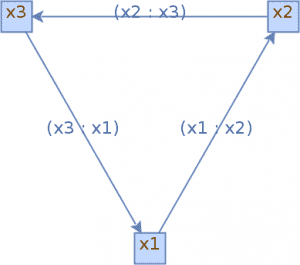We can find more details in the official documentation.

## 6. Conclusion

JGraphT provides almost all types of graphs and variety of graph algorithms. We covered how to use few popular APIs. However, you can always explore the library on the official page.

The implementation of all these examples and code snippets can be found over on Github.

### I just announced the new Learn Spring course, focused on the fundamentals of Spring 5 and Spring Boot 2:

>> CHECK OUT THE COURSE
Inline FeedbacksFrancois MAROT
3 years ago

Hi, thanks for the article. One remark though: you don’t show how to produce the images and I’m currently struggling finding out how to create png from in-memory graphs. It would be helpfull to add this code ! Thanks 🙂

3 years ago

Sounds like a good idea – I will add it to our backlog 🙂Francois MAROT
3 years ago

I you happen to have it near you, I would gladly take it. Could you copy-paste a few lines right here ? 🙂

3 years ago